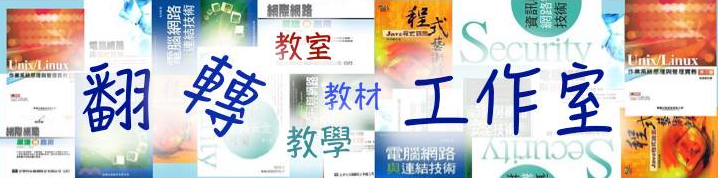Java 程式設計(一)  ：第 三章 算術運算式3-3 算術運算子

• 3-3-1 算術運算子彙集

• 3-3-2 範例研討：計算股票平均價格

• 3-3-3 自我挑戰：學期成績計算程式

• 3-3-4 範例研討：超商找錢工具

• 3-3-5 自我挑戰：超商收銀機系統

3-2 基本算術運算子

 運算符號 說明 +、- 正、負號 +、-、*、/、% 加、減、乘、除、模數（求餘數） ++、-- 遞增、遞減 ( …) 運算式集合

 x = x + y; 取出 x 與 y 兩變數內容，相『加』後再填入變數 x 內。 x = - x + y; 變數 x 內容取負數，與變數 y 相『加』後再填入變數 x 內。 x = x * y; 取出 x 與 y 兩變數內容，相『乘』後再填入變數 x 內。 z = x / y; 變數 x 除以 y，所得到的商存入變數 z 內。 z = x % y; 變數 x 除以 y，所得到的『餘數』存入變數 z 內；如 x = 5、y = 3，則 x % y 得到餘數為 2。 x++; 相當於 x = x+1; x-- 相當於 x = x – 1; x+= y; 相當於 x = x + y; x-= y; 相當於 x = x - y; x*= y; 相當於 x = x * y; x/= y; 相當於 x = x / y; x%= y; 相當於 x = x % y; (2 + 3) * (4 +5) 先處理括號內得到 5 * 9，最後結果為 45。

A）程式功能：Ex3_2.java

 *** 計算股票平均價系統 *** 請輸入第一個交易日股價 =>40.5 (目前平均價 = 40.50)請輸入第二個交易日股價 =>48.6 (目前平均價 = 44.55)請輸入第三個交易日股價 =>42.7 (目前平均價 = 43.93)請輸入第四個交易日股價 =>49.2 (目前平均價 = 45.25)請輸入第五個交易日股價 =>50.2 五日平均價 = 46.24

B）製作技巧研討：

C）程式範例：

 01 02 03 04 05 06 07 08 09 10 11 12 13 14 15 16 17 18 19 20 21 22 23 24 25 26 27 28 29 30 31 32 33 34 35 36 37 38 39 40 41 42 43 44 45 // Ex3_2.java import java.util.Scanner; public class Ex3_2 {        public static void main(String args[]) {         Scanner keyin = new Scanner(System.in);         float ave, sum=0, cost;         int number=0;         System.out.printf("*** 計算股票平均價系統 ***\n");         System.out.printf("請輸入第一個交易日股價 =>");         cost = keyin.nextFloat();         sum = sum + cost;         number++;         ave = sum / number;           System.out.printf("(目前平均價 = %.2f)請輸入第二個交易日股價 =>", ave);         cost = keyin.nextFloat();         sum = sum + cost;         number++;         ave = sum / number;                      System.out.printf("(目前平均價 = %.2f)請輸入第三個交易日股價 =>", ave);        cost = keyin.nextFloat();        sum = sum + cost;        number++;        ave = sum / number;          System.out.printf("(目前平均價 = %.2f)請輸入第四個交易日股價 =>", ave);        cost = keyin.nextFloat();        sum = sum + cost;        number++;        ave = sum / number;            System.out.printf("(目前平均價 = %.2f)請輸入第五個交易日股價 =>", ave);         cost = keyin.nextFloat();         sum = sum + cost;         number++;         ave = sum / number;         System.out.printf("五日平均價 = %.2f\n", ave);    } }

D）程式重點分析：

• 吾人可以發現計算 5 日平均價還稍可接受，如計算 102030 個交易日，程式將變得非常長，而大多書寫同樣的敘述句。因此，本系統應該使用迴圈敘述句，可容易許多，本書第五章將會介紹到。

• 7 ：『float ave, sum=0F, cost;』。表示同時宣告 3 個浮點變數，並給 sum 初值 0，但浮點數的數值必須多加 Fsum=0F）。

• 11 ：『sum = sum + cost;』。將 cost 內容累積增加到 sum 變數內。

• 12 ：『number++;』。功能是 number 變數累增 1number = number + 1）。

• 13 ：『ave = sum / number;』。計算當天之前連續交易日的平均股價。

A）程式功能：PM3_1.java

 *** 學期成績計算系統(各科學分數) *** 請輸入電腦概論成績(2 學分) =>80 請輸入程式設計成績(3 學分) =>87 請輸入離散數學成績(3 學分) =>72 請輸入國文成績(2 學分) =>65 請輸入英文成績(2 學分) =>74 學期總平均分數 = 76.25 四捨五入後成績 = 76

B）製作技巧提示：

 01 02 03 04 05 06 07 08 09 10 11 ……       System.out.printf("請輸入程式設計成績(3 學分) =>");       value = keyin.nextInt();       sum = sum + value * 3; ….. …..       ave = sum/12.0F;       System.out.printf("學期總平均分數 = %.2f\n", ave); ave = ave + 0.5F;       System.out.printf("四捨五入後成績 = %d\n", (int)ave); ….

A）程式功能：Ex3_3.java

 **** 超商找錢工具 **** 請輸入消費的金額 =>354 請輸入繳納金額（大於消費額 354） =>500 應找金額 = 146 各種零錢數量如下:          100 元零錢 = 1 張          50 元硬幣 = 0 個          10 元硬幣 = 4 個           5 元硬幣 = 1 個           1 元硬幣 = 1 個

B）製作技巧研討：

C）程式範例：

 01 02 03 04 05 06 07 08 09 10 11 12 13 14 15 16 17 18 19 20 21 22 23 24 25 26 27 28 29 30 31 32 33 34 35 36 37 // Ex3_3.java import java.util.Scanner; public class Ex3_3 {         public static void main(String args[]){         Scanner keyin = new Scanner(System.in);         int total, recept, value, value1, handre, fifty, ten, five, one;                 System.out.printf("**** 超商找錢工具 ****\n");         System.out.print("請輸入消費的金額 =>");         total = keyin.nextInt();         System.out.printf("請輸入繳納金額（大於消費額 %d） =>", total);         recept = keyin.nextInt();           value = recept - total;         value1 = value;         handre = value / 100;         value = value - handre * 100;           fifty = value / 50;         value = value - fifty * 50;           ten = value / 10;         value = value - ten * 10;           five = value / 5;         value = value - five * 5;           one = value;         System.out.printf("應找金額 = %d 各種零錢數量如下:\n", value1);         System.out.printf("\t 100 元零錢 = %d 張 \n", handre);         System.out.printf("\t 50 元硬幣 = %d 個 \n", fifty);         System.out.printf("\t 10 元硬幣 = %d 個 \n", ten);         System.out.printf("\t  5 元硬幣 = %d 個 \n", five);         System.out.printf("\t  1 元硬幣 = %d 個 \n", one);    } }

D）程式重點分析：

• 17 ：『hundre = value / 100;』。計算 100 元零錢的數目；整數相除僅得到整數，剩下的餘數則被取捨掉。

• 18 ：『value = value – hundre*100;』。計算找 100 元零錢數目後，剩下的找錢數目多寡；其實是求餘數的功能，讀者可將其改為 value = value % 100 執行看看，結果是否相同。

A）程式功能：PM3_2.java

 *****  超商收銀機系統  ***** 衛生紙(每包 32 元) 購買數量 =>3 口香糖(每包 12 元) 購買數量 =>5 可樂(每瓶 18 元) 購買數量 =>2 熱狗(每支 17 元) 購買數量 =>3 請輸入繳納金額(大於購買總金額 = 243) =>500 應找金額 = 257 各種零錢數量如下:          100 元零錢 = 2 張          50 元硬幣 = 1 個          10 元硬幣 = 0 個           5 元硬幣 = 1 個           1 元硬幣 = 2 個

B）製作技巧提示：

 01 02 03 04 05 06 07 08 09 10 11 12 13 14 …… ….         System.out.printf("*****  超商收銀機系統  *****\n");         System.out.printf("衛生紙(每包 32 元) 購買數量 =>");         item = in.nextInt();         total = total + item * 32;         ……                 System.out.printf("請輸入繳納金額(大於購買總金額 = %d) =>", total);         item = in.nextInt();         value = item - total;         value1 = value;        …..

Java 程式設計(一) 含程式邏輯